# TS Grewal Solution Class 12 Chapter 7 Death of a Partner

Read TS Grewal Accountancy Class 12 Solution Chapter 7 Death of a Partner 2022-23. Students should study TS Grewal Solutions Class 12 Accountancy available on Studiestoday.com with solved questions and answers. These chapter-wise answers for class 12 Accountancy have been prepared by the teacher of Grade 12. These TS Grewal class 12 Solutions have been designed as per the latest accountancy syllabus for class 12 and if practiced thoroughly can help you to score good marks in standard 12 Accounts class tests and examinations.

## Class 12 Accounts Chapter 7 Death of a Partner TS Grewal Solutions

TS Grewal Solutions for Chapter 7 Death of a Partner Class 12 Accounts have been provided below based on the latest TS Grewal Class 12 book. The answers have been prepared based on the latest 2022-2023 book for the current academic year. TS Grewal Solutions Class 12 will help students to improve their concepts and easily solve accountancy questions for class 12. Class 12 Grewal solutions should be revised regularly as more practice will help you get a better rank and easily solve more questions.

### Chapter 7 Death of a Partner TS Grewal Class 12 Solutions

TS Grewal Class 12 Chapter 7 Death of a Partner is a very important chapter if you have to understand the concepts of accounting to be done in the event if death of a partner of a partnership firm. The chapter mainly focuses on the dissolution of the partnership firm, and the settlement of accounts of the deceased partner, and the surviving partners. There are various important topics explained such as need for a partnership deed that clearly defines the terms and conditions of the partnership and actions to be taken when a partner dies, accounting aspects relating to sale of assets, settlement of liabilities, distribution of profits, and the settlement of the capital account of the deceased partner.

Along with the concepts there are detailed examples which have been provided in the TS Grewal book which will help the students to understand how to solve questions relating to this topic when they are asked in exams. There are lot of practical questions too. Our teachers have provided detailed solutions to all questions which will help them to score more marks in examinations.

Solutions for T.S. Grewal's Double Entry Book Keeping: Accounting for Not for Profit Organizations and Partnership Firms (Vol.1)
Textbook for CBSE Class 12
TS Grewal Solutions Class 12 Accountancy
Chapter 7
Death of a Partner

Question 1. Why heirs of retiring/deceased partner are entitled to share of goodwill of the firm?
The retiring partner/heirs of deceased partner are entitled to the share of goodwill because the goodwill earned by the firm is the result of the efforts of all the existing partners in the past.

Question 2. State the ratio in which the partners share gain or loss from revaluation of assets and liabilities.
The partners share gain or loss from revaluation of assets and liabilities in their old profitsharing ratio.

Question 3. How is the amount due to an outgoing partner dealt with in case it is not paid immediately?
Amount due to the retiring partner is determined by preparing Capital Account of the retiring partner.

Question 4. Name two adjustments to be made at the time of retirement or death of a partner.
Below are the adjustments to be made at the time of retirement or death of a partner:-
1.) Change in the profit-sharing Ratio i.e. determining New Profit-sharing Ratio and Gaining Ratio.
2.) Valuation and Adjustment of Goodwill.

Question 5. In which ratio do the remaining partners acquire the share of the deceased partner?
Gaining Ratio is necessary for acquire the share of the deceased partner.

Question 6. If the new profit-sharing ratio of remaining partners is not given, how is the share of the outgoing partner distributed?
If the new profit-sharing ratio of remaining partners is not given, then share of the outgoing partner distributed in old profit-sharing ratio.

Question 7. Name the account which is opened to credit the share of profit of the deceased partner, till the time of his death to his Capital Account.
Profit and Loss Suspense Account is opened to credit the share of profit of the deceased partner, till the time of his death to his Capital Account.

Question 8. State any two deductions that may have to be made from the amount payable to the legal representative of a deceased partner.
The legal representative of the deceased partner is eligible for the following amount:-
1.) The amount standing in the deceased partner’s capital A/c.
2.) The amount of interest on capital up to the date of death, if partnership deed so provides.

Question 9. At what rate is interest payable on the amount remaining unpaid to the executor of deceased partner?
In above case the executors of the deceased partners are entitled to interest @ 6% p.a. unless agreed otherwise.

Question 10. A, B and C are partners in a firm sharing profit and losses in the ratio 3:2:1. B died on 1st April, 2018. C, Son of B, is of the opinion that he is the rightful owner of his father’s share of profit, and the profit of the firm should be now shared between A and C equally. A does not agree. Settle the dispute between A and C by giving reason.

C is not correct in his claim, unless agreed; new profit-sharing ratio of the continuing partners remains same as their old profit – sharing ratio i.e. 3:1.

Question 1. What problems arise when a partner dies? How would you deal with them as an accountant?

Below are the problems arise when a partner dies:-

1.)   Change in profit sharing Ratio

2.)   Treatment of goodwill

3.)   Revaluation of assets and liabilities

4.)   Accumulated profit, reserve, losses etc.

5.)   Adjustment of Joint life Policy

Below adjustment should be deal with them as an accountant:-

1.)   Amount standing to the credit of the deceased Partner’s Capital Account and Current Account, if Capital Accounts are maintained following Fixed Capital Accounts Method.

2.)   His share in the goodwill of the firm.

3.)   His share of profit earned from the beginning of the financial year to the date of death.

4.)   His share of gain on revaluation of assets and reassessment of liabilities.

5.)   His share of accumulated profit and reserves.

6.)   Interest on capital up to the date of his death, if allowed by the Partnership Deed.

7.)   His salary or commission up to the date of his death, if allowed by the Partnership Deed.

Question 2. Explain the estimation of profit up to the date of death on the basis of time period involved.

It the time basis is used, the profit are assumed to have arisen uniformly over the year. Suppose, the profit for the previous year is Rs. 24,000 and a partner dies after tow months of the close of previous year. The profit for the two months will be Rs. 4,000 i.e. Rs 24,000 × . If the deceased partner 3/10th share of profit, his share of profit till the date of dearth will be Rs. 1200, the deceased partner’s share of profit is calculate das its agreed to among all the partner. It may be calculated on the basis of the provision year profit akledd it may be as or on basis of average profit of certain year.

EXERCISE..............

DEATH OF A PARTNER

Calculation of Profit of a Deceased Partner

Question 1:   Kumar, Verma and Naresh were partners in a firm sharing profits and Loss in the ratio of 3 : 2 : 2 . On 23rd January, 2015 Verma died. Verma's share of profit till the date of his death was calculated at Rs 2,350. Pass necessary journal entry for the same in the books of the firm.

The Journal entry for transferring Verma’s share of profit to his capital account is given below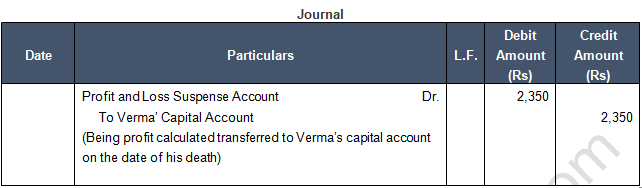Question 2:   A, B and C were partners sharing profits and losses in the ratio of 2 : 2 : 1. C died on 30th June, 2018. Profit and Sales for the year ended 31st March, 2018 were Rs 1,00,000 and Rs 10,00,000 respectively. Sales during April to June, 2018 were Rs 1,50,000. You are required to calculate share of profit of C up to the date of his death.

The share of profit of C up to the date of deathQuestion 3:  A, B and C are partners sharing profits and losses in the ratio of 3 : 2 : 1 .B died on 30th June, 2018. For the year ended 31st March, 2019, proportionate profit of 2018 is to be taken into consideration. During the year ended 31st March, 2018, bad debts of Rs 2,000 had to be adjusted. The profit for the year ended 31st March, 2018 was Rs 14,000 before adjustment of bad debts. Calculate B's share of profit till the date of his death.

B’s share of profit till his deathQuestion 4:  Ram, Manohar and Joshi were partners in a firm. Joshi died on 31st May, 2018. His share of profit from the closure of the last accounting year till the date of death was to be calculated on the basis of the average of three completed years of profits before death. Profits for the years ended 31st March, 2016, 2017 and 2018 were Rs 7,000; Rs 8,000 and Rs 9,000 respectively. Calculate Joshi's share of profit till the date of his death and pass necessary journal entry for the same.

Joshi’s share of profit from the closure of the last accounting year till the date of death
{Average Profit × Number of Months worked till the date of death /12 Months} × Joshi’s share of profit
= (7,000+8,000+9,000) × 2/12 × 1/3 = 445 ApproximatelyQuestion 5:  X, and Z were partners sharing profits and losses in the ratio of 3 : 2 : 1 respectively. Y died on 30th June, 2018. The Profit from 1st April, 2018 to 30th June, 2018 amounted to Rs 3,60,000. X and Z decided to share the future profits in the ratio of 3 : 2 respectively with effect  from 1st July, 2018 . Pass the necessary journal entries to record Y's share of profit up to the date of death.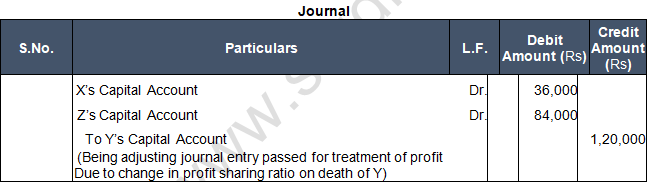Points of Knowledge:

Old Ratio of X:Y:Z = 3:2:1

New Ratio of X:Z = 3:2

Gaining Ratio = New Ratio – Old Ratio

X’s Gain = New Share – Old Share = 3/5 - 3/6 = (18-15)/30 = 3/30

Z’s Gain = New Share – Old Share = 2/5 - 1/6 = (12-5)/30 = 7/30

Gaining Ratio of X:Z = 3:7

Profit earned = 3,60,000

Y’s share of profits till the date of death

= 3,60,000 × 2/6 = 1,20,000

Y’s Capital Account credited for profit = 1,20,000

X’s Capital Account debited for profit

= 1,20,000 × 3/10 = 36,000

Z’s Capital Account debited for profit

= 1,20,000 × 7/10 = 84,000

Condition for profit treatment: Gaining Partner to Sacrificing Partner.

Question 6:  X, Y and Z were partners in a firm. Z died on 31st May, 2018. His share of profit from the closure of the last accounting year till the date of death was to be calculated on the basis of the average of three completed Rs 19,000 and Rs 17,000 respectively.

Calculate Z's share of profit  till his death and pass  necessary journal entry for the same assuming:
(a) there is no change in profit-sharing ratio of remaining  partners, and
(b) there is change in profit-sharing ratio of remaining partners, new ratio being 3 : 2 .Note 2 : Old Ratio of X:Y:Z = 1:1:1
New ratio of X : Y = 3 : 2
Gaining Ratio = New Ratio – Old Ratio
X’s Gain = New Share – Old Share = 3/5 - 1/3 = (9-5)/15 = 4/15
Y’s Gain = New Share – Old Share = 2/5 - 1/3 = (6-5)/15 = 1/15
Gaining Ratio of X : Y = 4 : 1
Average Profit = (Total Profit)/(Total Years) = ((18,000+19,000+17,000))/3 = 18,000
Z’s share of profit credited to his capital Account
= 18,000 × 1/3 × 2/12 = 1,000
X’s Capital Account debited for profit = 1,000 × 4/5 = 800
Y’s Capital Account debited for profit = 1,000 × 1/5 = 200

Deceased Partner’s Share of Goodwill

Question 7:  P, R and S are in partnership sharing profits 4/8, 3/8 and 1/8 respectively. It is provided in the Partnership Deed that on the death of any partner his share of goodwill is to be valued at one-half of the net profit credited to his account during the last four completed years.

R died on 1st January, 2018. The firm’s profits for the last four years ended 31st December, were as:
2014——Rs 1,20,000; 2015——Rs 80,000; 2016——Rs 40,000; 2017——Rs 80,000.
(a) Determine the amount that should be credited to R in respect of his share of Goodwill.
(b) Pass journal entry without raising Goodwill Account for its adjustment .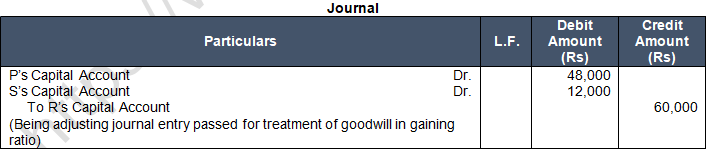Points of Knowledge:

Note 1: Calculation of Gaining Ratio
Old Ratio of P : R : S = 4 : 3 : 1
New Ratio of P : S = 4 : 1
Gaining Ratio = New Ratio – Old Ratio
P’s Gain = 4/5 - 4/8 = (32-20)/40 = 12/40
S’s Gain = 1/5 - 1/8 = (8-5)/40 = 3/40
Therefore, Gaining Ratio of P:S = 12:3 = 4:1
(a) Amount that should be credited to R in respect of his share of Goodwill
= R’s share of goodwill is to be valued at one half of the net profit credited to his account during the last four completed years
= (1,20,000+80,000+40,000+80,000) × 3/8 × 1/2 = Rs. 60,000
P will transfer for goodwill to R = 60,000 × 4/5 = Rs. 48,000
S will transfer for goodwill to R = 60,000 × 1/5 = Rs. 12,000

Question 8:  X, Y and Z were partners in a firm sharing profit in 3 : 2 : 1 ratio. The firm closes its books on 31st March every year. Y died on 30th June, 2018. On Y's death the goodwill of the firm was valued at Rs60,000. Y's share in the profits of the firm till the time of his death was to be calculated on the basis of previous year's profit which was Rs 1,50,000.

Pass necessary journal entries for the treatment of goodwill and Y's share of profit at the time of his death.Note 1: Calculation of Gaining Ratio
Old Ratio of X : Y : Z = 3 : 2 : 1
New Ratio of X : Z = 3 : 1
Gaining Ratio = New Ratio – Old Ratio
X’s Gain = 3/4 - 3/6 = (18-12)/24 = 6/24
Z’s Gain = 1/4 - 1/6 = (6-4)/24  = 2/24
Therefore Gaining Ratio of X : Z = 6 : 2 = 3 : 1
Goodwill of the firm = 60,000
Y’s share of goodwill = 60,000 × 2/6 = 20,000
X’s Capital Account debited for goodwill = 20,000 × 3/4 = 15,000
Z’s Capital Account debited for goodwill = 20,000 × 1/4 = 5,000
Y’s share of profit till the date of his death = 1,50,000 × 3/12 × 2/6 = 12,500

Question 9:  A, B and C were partners sharing profits in the ratio of 3 : 2 : 1. The firm closes its books on 31st March every year. B died on 30th June, 2018. On his death, Goodwill of the firm was valued at Rs. 6,00,000. B's share in profit or loss till the date of death was to be calculated on the basis of previous year's profit which was Rs. 15,00,000 (Loss). Pass necessary Journal entries for goodwill and his share of loss.Point of Knowledge:-

Calculation of B’s Share of Goodwill
Total Goodwill of Firm = 6,00,000
B’s Share of Goodwill = 6,00,000 × 2/6 = Rs. 2,00,000

Distribution of Goodwill
A’s Share of Goodwill = 2,00,000 × 3/4 = Rs. 1,50,000
C’s Share of Goodwill = 2,00,000 × 1/4 = Rs. 50,000

Calculation of B’s Share of Loss:-
Total Loss = Rs. 15,00,000
B’s Share of loss = 15,00,000 × 2/6 × 3/12 (months till date of his death)
= 1,25,000

Deceased Partner’s Share of Goodwill and Profit

Question 10:  X, Y and Z were partners in a firm sharing profits in the ratio of 4 : 3 : 1 . The firm closes its books on 31st March every year. On 1st February, 2019, Y died and it was decided that the new profit-sharing ratio between X and Z will be equal. Partnership Deed provided for the following on the death of a partner:

(a)   His share of goodwill be calculated on the basis of half of the profits credited to his account during the previous four completed years. The firm's profits for the last four years were: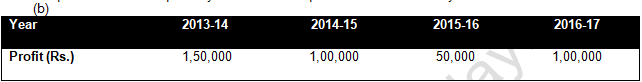(c)   His share of profit in the year of his death was to be computed on the basis of average profit of past two years.

Pass necessary journal entries relating to goodwill and profit to be transferred to Y's Capital Account.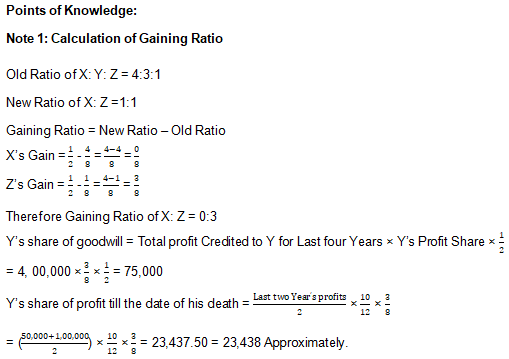Determination of Amount Payable to Executors of a Deceased Partner

Question 11:  X and Y are partners. The Partnership Deed provides inter alia:

(a) That the Accounts be balanced on 31st March every year.

(b) That the profits be divided as: X one-half, Y one-third and carried to a Reserve one-sixth.

(c) That in the event of the death of a partner, his Executors be entitled to be paid out:

i.        The Capital to his credit till the date of death.

ii.        His proportion of profits till the date of death based on the average profits of the last three completed years.

iii.        By way of Goodwill, his proportion of the total profits for the three preceding years.The Profits for three years were : 2015-16——Rs 4,200; 2016-17——Rs  3,900; 2017-18——Rs  4,500. Y died on 1st August, 2018. Prepare necessary accounts.Points of Knowledge:

Note 1: Calculation of Ratio

Old Ratio of X:Y:Reserve = ½:1/3:1/6
= 1/2×3/3:1/3×2/2:1/6
= 3/6:2/6:1/6
= 3:2:1
Old Ratio of X:Y = 3:2
After Y’s death the share of X will be 1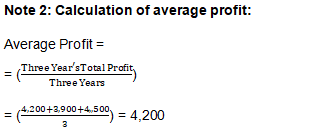Note 3 : Goodwill of the firm = Three years Total Profit

= 4,200+3,900+4,500 = 12,600

Y’s share of Goodwill = 12,600 × 2/5 = 5,040

Note 4 : Y’ share of profit = Average Profit × 4/12 Months × 2/5

= 4,200 × 4/12 × 2/5 = 560

Note 5 : Y’s share of reserve = 3,000 × 2/5 = 1,200

Question 12:  P, Q and R were partners in a firm sharing profits in 2 : 2 : 1 ratio. The Partnership Deed provided that on the death of a partner his executors will be  entitled to the following:

(a) Interest on Capital @ 12% p.a.
(b) Interest on Drawings @ 18% p.a.
(c) Salary of Rs 12,000 p.a.
(d) Share in the profit of the firm( up to the date of death) on the basis of previous year's profit.
P died on 31st May, 2108. His capital was Rs 80,000. He had withdrawn Rs 15,000 and interest on his drawings was calculated as Rs 1,200. Profit of the firm for the previous year ended 31st March, 2018 was Rs 30,000.
Prepare P's Capital Account to be rendered to his executorsPoints of Knowledge:

(a) Interest on P’s Capital @12% p.a. till the date of death

= 80,000 × 12/100 × 2/12 = 1,600

(b) P’s share of profits till the death calculated on the basis of last year’s profits

= 30,000 × 2/12 × 2/5 = 2,000

(c) P’s salary = 12,000 × 2/12 = 2,000

Question 13:  Vikas, Gagan and Momita were partners in a firm sharing profits in the ratio of 2 : 2 : 1 . The firm closes its books on 31st March every year. On 30th September, 2014 Momita died. According to the provisions of Partnership Deed the legal representatives of a deceased partner are entitled for the following in the event of his/her death:

(a) Capital as per the last Balance Sheet.

(b)  Interest on capital at 6% per annum till the date of her death.

(c) Her share of profit to the date of death calculated on the basis of average profit of last four years.
(d) Her share of goodwill to be determined on the basis of three years' purchase of the average profit of last four years. The profits of last four years were;The balance in Momita's Capital Account on 13st March, 2014 was Rs. 60,000 and she had withdrawn Rs. 10,000 till date of her death. Interest on her drawings was Rs. 300. Prepare Momita's Capital Account to be presented to her executors.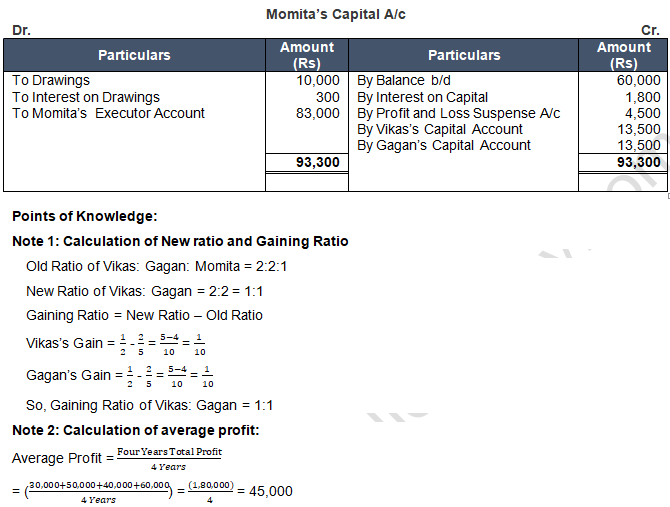Note 3 : Goodwill of the firm = Four Years Average Profit × Three Years Purchase
= 45,000 × 3 = 1,35,000
Momita will get for goodwill from Vikas and Gagan = 1,35,000 × 1/5 = 27,000
Vikas will transfer for goodwill to Momita = 27,000 × 1/2 = 13,500
Gagan will transfer for goodwill to Momita = 27,000 × 1/2 = 13,500
Condition for goodwill treatment: Remaining Partners to Dead Partners

Note 4 : Calculation of Profit to be given to the dead partners Representatives
Momita’s share of profit = Average Profit × 6/12 Months × 1/5
= 45,000 × 6/12 Months × 1/5 = 4,500

Note 5: Interest on Capital = 60,000 × 6/12 Months × 6/100 = 1,800

Question 14:  Iqbal and Kapoor are in partnership sharing profits and losses in 3 : 2 . Kapoor died three months after the date of the last Balance Sheet. According to the Partnership Deed, the legal personal representatives of Kapoor are entitled to the following payments:

(a) His capital as per the last Balance Sheet.

(b) Interest on above capital @ 3% p.a. till the date of death.

(c) His share of profits till the date of death calculated on the basis of last year's profits.

His drawings are to bear interest at an average rate of 2% on the amount irrespective of the period. The net profits for the last three years, after charging insurance premium, were Rs 20,000; Rs 25,000 and Rs 30,000 respectively. Kapoor's capital as per Balance Sheet was Rs 40,000 and his drawings till the date of death were Rs 5,000.

Draw Kapoor's Capital Account to be rendered to his representatives.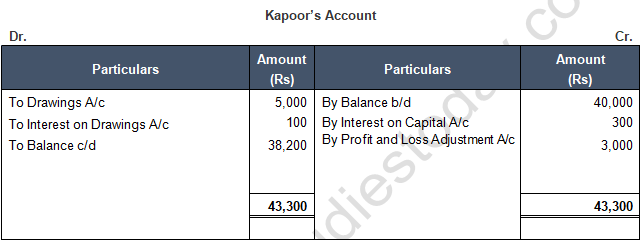Points of Knowledge:

(a)   Interest on Kapoor’s Capital @ 3% p.a. till the date of death.

= 40,000 × 3/100 × 3/12 = 300

(b)   Kapoor’s Share of profits till the date of death calculated on the basis at last year’s profits

= 30,000 × 3/12 × 2/5 = 3,000

(c)   Interest on Kapoor’s Drawings @ 2% irrespective of time period

= 5,000 × 2% = 100

Question 15:  A, B and C were partners in a firm sharing profits in the ratio of 5 : 3 ; 2 . On 31st March, 2017, their Balance Sheet was as follows:A died-on 1st October, 2017. It was agreed  among his executors and the remaining partners that:
(i) Goodwill to be valued at 212/212 years' purchase of the average  profit of the previous 4 years , which were 2013-14: Rs  13,000; 2014-15: Rs  12,000; 2015-16: Rs  20,000 and 2016-17: Rs  15,000.
(ii) Patents be valued at Rs  8,000; Machinery at Rs  28,000; and Building at Rs  25,000.
(iii) Profits for the year  2017-18 be taken as having  accrued at the same rate as that of the previous year .
(iv) Interest on capital  be provided @ 10% p.a.
(v) Half of the amount due to A to be paid immediately to the executors  and the balance transferred to his ( Executors) Loan Account.
Prepare A's Capital Account and A's Executors Account as  on 1st October, 2017.Points of Knowledge:

Note 1: Calculation of New Ratio and Sacrificing Ratio:
Old Ratio of A:B:C = 5:3:2
New Ratio of B:C = 3:2
Gaining Ratio = New Ratio – Old Ratio
B’s Gain = 3/5 - 3/10 = (6-3)/10 = 3/10
C’s Gain = 2/5 - 2/10 = (4-2)/10 = 2/10
So Gaining Ratio of B:C = 3:2Note 3 : Goodwill of the firm

= Average Profit × Two and half Years Purchase
= 15,000 × 2.5 = 37,500
A will get for goodwill from B and C
= 37,500 × 5/10 = 18,750
B will transfer for goodwill to A
= 18,750 ×  3/5 = 11,250
C will transfer for goodwill to A
= 18,750 × 2/5 = 7,500
Condition for goodwill treatment: Gaining Partner to Dead Partner

Note 4 :
Calculation of profit to be given to the dead partners Representatives
A’s share of profit
= Last Year Profit × 6/12  Months × 5/10
= 15,000 × 6/12  × 5/10 = 5,000

Note 5 : Interest on Capital
= 25,000 × 10% × 6/12 = 1,250

Note 6 : There is no revaluation profit or loss

Question 16:  Virad, Vishad and Roma were partners in a firm sharing profits in the ratio of 5 : 3 : 2 respectively. On 31st March, 2103, their Balance Sheet was as under: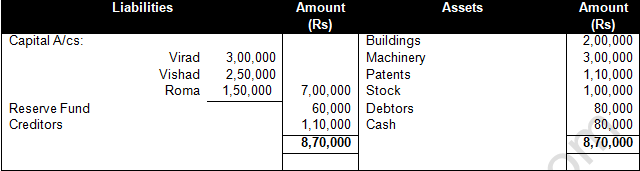Virad died on 1st October, 2013. It was agreed between his executors and the remaining partners that:

(i) Goodwill of the firm be valued at 212/212 years purchase of average profits for the last three years. The average profits were Rs. 1,50,000.
(ii) Interest on capital be provided at 10% p.a.
(iii) Profits for the 2013-14 be taken as having accrued at the same rate as that of the previous year which was Rs.1,50,000.
Prepare Virad's Capital Account to be presented to his Executors as on 1st October, 2013.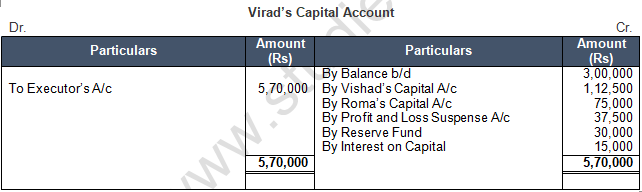Question 17:  Kavita, Leena and Monica are partners in firm sharing profits in the ratio of 1 : 1 : 3 respectively. Their Capital Accounts showed the following balances on 31st March, 2012: Kavita Rs 70,000; Leena Rs 65,000 and Monica Rs 2,10,000. Firm closes its accounts every year on 31st March. Kavita died on 30th September, 2012. In the event of death of any partner, the Partnership Deed provides for the following :

(a) Interest on capital will be calculated at the rate of 6% p.a.

(b) The deceased partner's share in the goodwill of the firm will be calculated on the basis of 2 years' purchase of the average profit of last three years. The profits of the firms for the last three years were Rs 90,000; Rs 1,00,000 and Rs 1,10,000 respectively.

(c) Her share in the Reserve Fund of the firm will be paid. The Reserve Fund of the firm was Rs 60,000 at the time of Kavita's death.

(d) Her share of profit till the date of death will be calculated on the basis of sales. It is also specified that the sales during the year 2011-12 were Rs 20,00,000. The sales from 1st April, 2012 to 30th September, 2012 were Rs 4,00,000. The profit of the firm for the year ending 31st March, 2012 was Rs 2,00,000.
Prepare Kavita's Capital Account to be presented to his legal representative.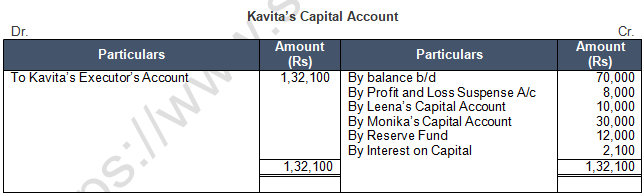Points of Knowledge :

Note 1 : Calculation of New Ratio and Gaining Ratio

Old Ratio of Kavita:Lenna:Monika = 1:1:3
New Ratio of Lenna:Monika =1:3
Gaining Ratio = New Ratio – Old Ratio
Lenna’s Gain = 1/4 - 1/5 = (5-4)/20 = 1/20
Monika’s Gain = 3/4 - 3/5 = (15-12)/20 = 3/20
So Gaining Ratio of Lenna: Monika = 1:3Question 17:  A, B and C are partners in a firm sharing profits in the proportion of 3 : 2 : 1 . Their Balance Sheet as at 31st March, 2018 stood as follows:died on 30th June , 2018 and according to the deed of the said partnership his executors are entitled to be paid as under:
(a) The capital to his credit at the time of his death and interest thereon @ 10% per annum.
(b) His proportionate share of General Reserve.
(c) His share of profits  for the intervening period will be based on the sales during that period. Sales from 1st April, 2018 to 30th June, 2018 were as Rs 12,00,000. The rate of profit during past three years had been 10% on sales.
(d) Goodwill according to his share of profit to be calculated by taking twice the amount of profits of the last three years less 20%. The profit of the previous three years were: 1st Year: Rs. 82,000; 2nd year: Rs 90,000; 3rd year Rs 98,000.
(e) The investments were sold at par and his executors were paid out in full.
Prepare B's Capital Account and his Executors' Account.Points of Knowledge:

Note 1: Calculation of New Ratio and Sacrificing Ratio
Old Ratio of A:B:C = 3:2:1
New Ratio of A:C = 3:1
Gaining Ratio = New Ratio – Old Ratio
A’s Gain = 3/4 - 3/6 = (18-12)/24 = 6/24
C’s Gain = 1/4 - 1/6 = (6-4)/24 = 2/24
So Gaining Ratio of A:C = 6:2 = 3:1Note 3: Goodwill of the firm = Three years Total Profit × Two times Purchase – 20%
= 2,70,000 × 2 – 5,40,000 × 20% = 4,32,000
B will get for goodwill from A and C = 4,32,000 × 2/6 = 1,44,000
A will transfer for goodwill to B = 1,44,000 × 3/4 = 1,08,000
C will transfer for goodwill to B = 1,44,000 × 1/4 = 36,000

Note 4: Condition for goodwill treatment: Remaining Partners to Dead partner
B’s share of profit = Sales during the period × % of Normal Profit × B’s Profit share
= 12,00,000 × 10/100 × 2/6 = 40,000

Note 5: Interest on Capital = 1,20,000 × 10% × 3/12 = 3,000

Question 18:   Babita, Chetan and David are partners in a firm sharing profits in the ratio of 2 : 1 : 1 respectively. Firm closes its accounts on 31st March every year. Chetan died on 30th September, 2012. There was a balance of Rs 1,25,000 in Chetan's Capital Account in the beginning of the year. In the event of Death of any partner, the Partnership Deed provides for the following:

(a) Interest on capital will be calculated at the rate of 6% p.a.

(b) The executor of deceased partner shall be paid Rs 24,000 for his share of goodwill.

(c) His share of Reserve Fund of Rs 12,000, shall be paid to his executor.

(d) His share of profit till the date of death will be calculated on the basis of sales. It is also specified that the sales during the year 2011-12 were Rs 4,00,000. The sales from 1st April, 2012 to 30th September, 2012 were Rs 1,20,000. The profit of the firm for the year ending 31st March, 2012 was Rs 2,00,000. Prepare Chetan's Capital Account to be presented to his executor.Points of Knowledge:

Note 1: Calculation of new Ratio and Gaining Ratio
Old Ratio of Babita:Chetan:David = 2:1:1
New Ratio of Babita:David = 2:1
Gaining Ratio = New Ratio – Old Ratio
Babita’s Gain = 2/3 - 2/4 = (8-6)/12 = 2/12
David’s Gain = 1/3 - 1/4 = (4-3)/12 = 1/12
So Gaining Ratio of Babita:David = 2:1

Note 2 :
Chetan will get for goodwill = 24,000
Babita will transfer for goodwill to Chetan = 24,000 × 2/3 = 16,000
David will transfer for goodwill to Chetan = 24,000 × 1/3 = 8,000
Condition for goodwill treatment: Remaining Partners to Dead partner.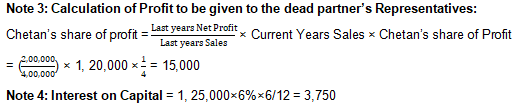Question 19:  Sunny, Honey and Rupesh were partners in a firm. On 31st March, 2014, their Balance Sheet was as follows: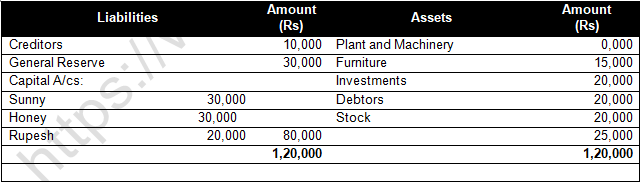Honey died on 31st December, 2014. The Partnership Deed provided that the representative of the deceased partner shall be entitled to :
(a) Balance in the Capital Account of the deceased partner.
(b) Interest on Capital @ 6%  per annum up to the date of his death.
(c) His share in the undistributed profits or losses  as per the Balance Sheet.
(d) His share in the profits of the firm till the date of his death , calculated on the basis of rate of net profit  on sales of the previous yea . The rate of net profit on sales of previous year was 20%. Sales of the firm during the year till 31st December, 2014 was Rs 6,00,000.
Prepare Honey's Capital Account to be presented to his executors.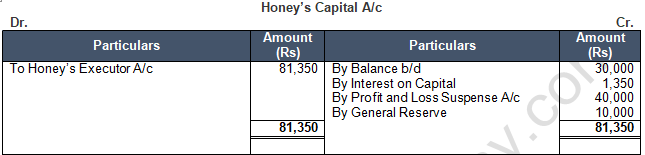Points of Knowledge:

Note1: Calculation of New Ratio

Old Ratio of Sunny: Honey: Rupesh = 1: 1: 1

New Ratio of Sunny: Rupesh = 1:1

Note2: Calculation of Profit to be given to the dead partner’s Representatives:

Honey’s share of profit = Sales during the Period × % of Normal Profit × Honey’s Profit Share

= 6, 00,000 × 20/100 × 1/3 = 40,000

Question 20:  R, S and T were partners sharing profits and losses in the ratio of 5 : 3 : 2 respectively. On 31st March, 2018, Their Balance Sheet stood as:T died on 1st August , 2018 . It was agreed that:
(a) Goodwill be valued at 212/212 years' purchase of average of last 4 years' profits which were:
2014-15: Rs 60,000; 2016-17: Rs 80,000 and 2017-18: Rs 75,000.
(b) Machinery be valued at Rs 1,40,000; Patents  be valued at  Rs 40,000;  Leasehold be valued at Rs 1,25,000 on 1st August, 2018..
(c) For the purpose of calculating T's share in the profits of 2018-19, the profits in 2018-19 should be taken to have accrued on the same scale  as in 2017-18.
(d) A sum of Rs 21,000 to be paid immediately to the Executors of T and the balance to be paid in four equal half-yearly instalments together with interest @ 10% p.a.
Pass necessary journal entries  to record the above transactions  and T's  Executors ' Account .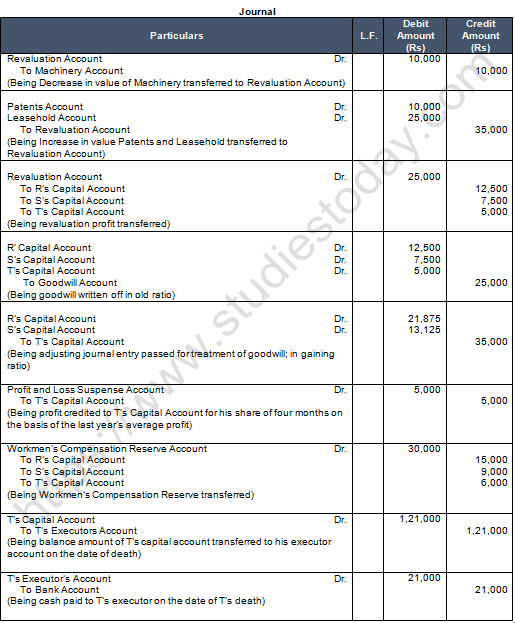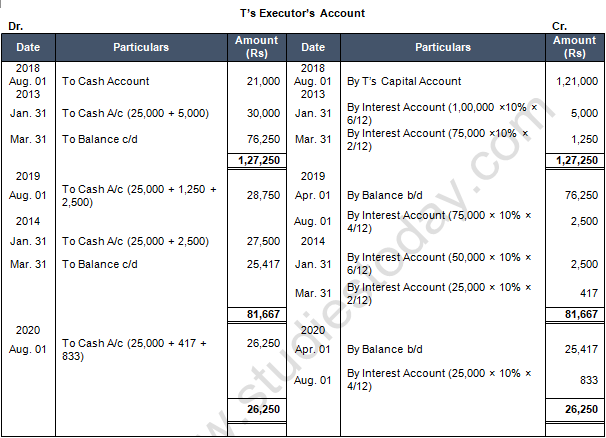Points of Knowledge:

Note 1 : Calculation of New Ratio and Gaining Ratio
Old Ratio of R:S:T = 5:3:2
New Ratio of R:S = 5:3
Gaining Ratio = New Ratio – Old Ratio
R’s Gain = 5/8 - 5/10 = (50-40)/80 = 10/80
S’s Gain = 3/8 - 3/10 = (30-24)/80 = 6/80
So Gaining Ratio of R:S = 5:3Note 3: Goodwill of the firm = Four years Average Profit × Two and Half Years Purchase
= 70,000 × 2.5 = 1,75,000
T will get for goodwill from R and S = 1,75,000 × 2/10 = 35,000
R will transfer for goodwill to T = 35,000 ×5/8 = 21,875
S will transfer for goodwill to T = 35,000 × 3/8 = 13,125
Condition for goodwill treatment: Remaining Partners to Dead partner
Note 4: Calculation of Profit to e given to the dead partners Representatives
T’s share of profit = Last Year’s Profit × 4/12 Months × 2/10
= 75,000 × 4/12 × 2/10 = 5,000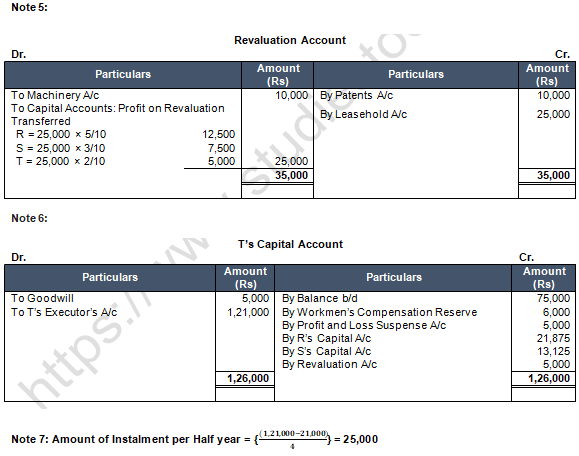Question 21:   Akhil, Nikhil and Sunil were partners sharing profits and losses equally. Following was their Balance Sheet as at 31st March, 2018: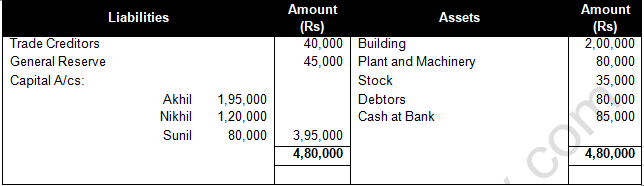Sunil died on 1st August, 2018. The Partnership Deed provided that the executor of a deceased partner was entitled to :
(a) Balance of Partners' Capital Account and his share  of accumulated reserve.
(b) Share of profits from the closure of the last accounting year till the date of death on the basis of the profit of the preceding completed year before death.
(c) Share of goodwill calculated on the basis of three times the average profit of the last four years.
(d) Interest on deceased partner's capital @ 6% p.a.
(e) Rs 50,000 to be paid to deceased's executor immediately and the balance to remain in his Loan Account.
Profits and Losses for the preceding years were: 2014-15——Rs 80,000 Profit; 2015-16——Rs 1,00,000 Loss; 2016-17——Rs 1,20,000 Profit; 2017-18——Rs 1,80,000 Profit.
Pass necessary journal entries and prepare Sunil's Capital Account  and Sunil's Executor Account .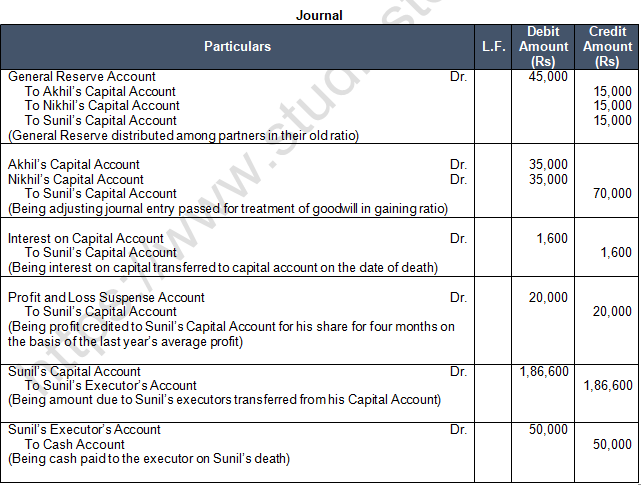Points of Knowledge:

Note 1: Calculation of New Ratio and Gain Ratio:-
Old Ratio of Akhil:Nikhil:Sunil = 1:1:1
New Ratio of Akhil:Nikhil = 1:1
Gaining Ratio = New Ratio – Old Ratio
Akhil’s Gain = 1/2 - 1/3 = (3-2)/6 = 1/6
Nikhil’s Gain = 1/2 - 1/3 = (3-2)/6 = 1/6
So Gaining Ratio of Akhil:Nikhil = 1:1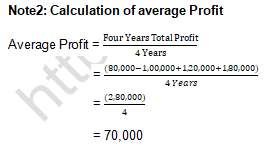Note 3 : Goodwill of the firm = Four years Average Profit ×Three Years Purchase:-
= 70,000 × 3 = 2,10,000
Sunil will get for goodwill from Akhil and Nikhil
= 2,10,000 × (1 )/3 = 70,000
Akhil will transfer for goodwill to Sunil
= 70,000 × 1/2 = 35,000
Nikhil will transfer for goodwill to Sunil
= 70,000 ×  1/2 = 35,000
Condition for goodwill treatment: Remaining Partners to Dead partner.

Note 4: Calculation of Profit to be given to the dead partners Representatives:-
Sunil’s share of profit
= Last Year’s Profit × 4/12 Months × 1/3
= 1,80,000 × 4/12× 1/3 = 20,000

Question 22:   B, C and D were partners in a firm sharing profits in the ratio of 5 :3 : 2 . On 31st December, 2008, their Balance Sheet was as follows:died on 31st March, 2009. The Partnership Deed provided for the following on the death of a partner:
(a) Goodwill of the firm was to be valued at 3 years' purchase of the average profit of last 5 years. The profits for the years ended 31st December, 2007, 31st December 2006, 31st December 2005, and 31st December 2004 were Rs 70,000 ; Rs 60,000 and Rs 40,000 respectively.
(b) B's share of profit and loss till the date of his death was to be calculated on the basis of the profit and loss for the year ended  31st December, 2008.
You are required to calculate the following :
(i) Goodwill of the firm and B's share of goodwill at the time of his death.
(ii) B's share in the profit or loss of the firm till the date of his death .
(iii) Prepare B's Capital Account at the time of his death  to be presented to his Executors .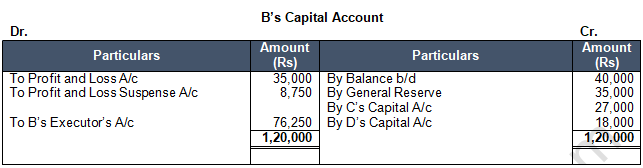Points of Knowledge:

Note 1: Calculation of New Ratio and Gaining Ratio:-
Old Ratio of B:C:D = 5:3:2
New Ratio of C:D = 3:2
Gaining Ratio = New Ratio – Old Ratio
C’s Gain = 3/5 - 3/10 = (6-3)/10 = 3/10
D’s Gain = 2/5 - 2/10 = (4-2)/10 = 2/10
So Gaining Ratio of C:D = 3:2
Condition for goodwill treatment: Remaining Partners to Dead partner:-

Note 3: Goodwill of the firm = Five years Average Profit × Three Years Purchase
= 30,000 × 3 = 90,000
B will get for goodwill from C and D
= 90,000 ×5/10= 45,000
C will transfer for goodwill to B
= 45,000 × 3/5 = 27,000
D will transfer for goodwill to B
= 45,000 × 2/5 = 18,000

Note 4: Calculation of Profit to be given to the dead partners Representatives:
B’s share of Loss
= Last Year’s Loss × 3/12× 5/10
= 70,000 × 3/12 × 5/10 = 8,750

Note 5: B’s share of Last Year’s Loss
= 70,000 × 5/10 = 35,000

Question 23:  The Balance Sheet of X, Y and Z as at 31st March, 2018 was: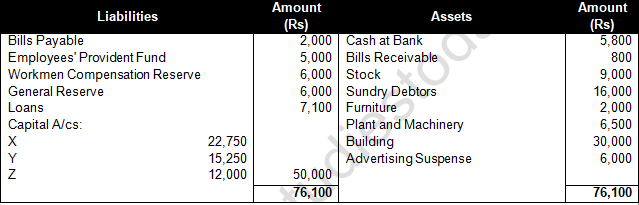The profit-sharing ratio was 3 : 2 : 1 . Z died on 31st July, 2018 . The Partnership Deed provides that:

(a) Goodwill is to be calculated on the basis of three years' purchase of the five years' average profit. The profits were: 2017-18: Rs 24,000; 2016-15: Rs 20,000; 2014-15: Rs 10,000 and 2013-14: Rs 5,000.
(b) The deceased partner to be given share of profits till the date of death on the basis of profits for the previous year.

(c) The Assets have been revalued as: Stock Rs. 10,000; Debtors Rs 15,000; Furniture Rs 1,500; Plant and Machinery Rs 5,000; Building Rs 35,000. A Bill Receivable for Rs 600 was found worthless.

(d) A Sum of Rs 12,233 was paid immediately to Z's Executors and the balance to be paid in two equal annual instalments together with interest@ 10% p.a. on the amount outstanding .
Give journal entries and show the Z's Executors' Account till it is finally  settled .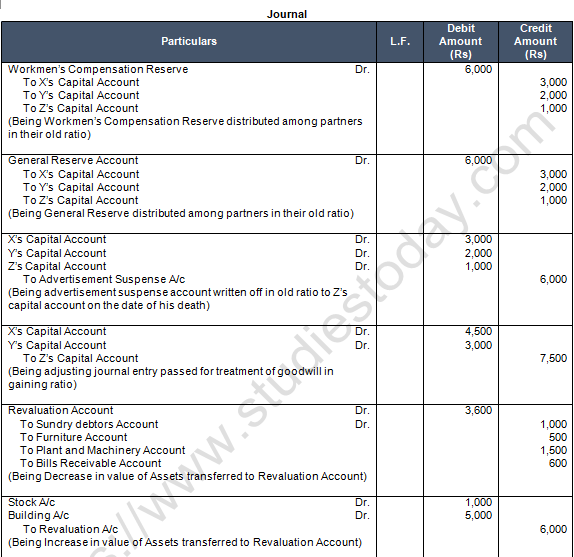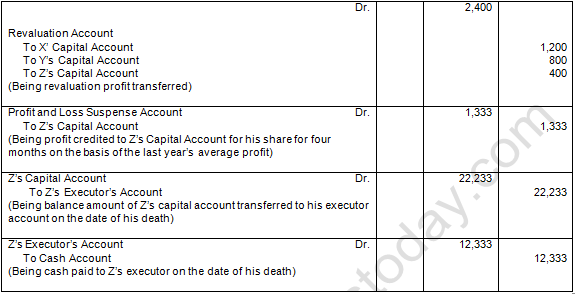Points of Knowledge :

Note 1: Calculation of New Ratio and Gaining Ratio

Old Ratio of X:Y:Z = 3:2:1
New Ratio of X:Y = 3:2
Gaining Ratio = New Ratio – Old Ratio
X’s Gain = 3/5 - 3/6 = (18-15)/30 = 3/30
Y’s Gain = 2/5 - 2/6 = (12-10)/30 = 2/30
So Gaining Ratio of X:Y = 3:2Note 3: Goodwill of the firm = Five years Average Profit × Three Years Purchase
= 15,000 × 3 = 45,000
Z will get for goodwill from X and Y
= 45,000 × 1/6 = 7,500
X will transfer for goodwill to Z
= 7,500 × 3/5 = 4,500
Y will transfer for goodwill to Z
= 7,500 ×2/5 = 3,000
Condition for goodwill treatment: Remaining Partners to Dead partner.

Note 4: Calculation of Profit to be given to the dead partners Representatives:
Z’s share of Profit
= Last Year’s Profit × 4/12 Months × 1/6
= 24,000 × 4/12 × 1/6 = 1,333Question 24:   X, Y and Z were partners in a firm sharing profits and losses in the 5 ; 4 : 3 . Their Balance Sheet on 31st March, 2018 was as follows:X died on 1st October, 2018 and Y and Z decide to share future profits in the ratio of 7 : 5 . It was agreed between his executors and the remaining partners that :
(i) Goodwill of the firm be valued at 212/212 years' purchase of average of four completed years' profit which were:(ii) X's share of profit from the closure of last accounting year till date of death be calculated on the basis of last years' profit.
(iii) Building undervalued by Rs 2,00,000;  Machinery overvalued by Rs 1,50,000 and Furniture overvalued by Rs 46,000.
(iv) A provision of 5% be created on Debtors for Doubtful Debts.
(v) Interest on Capital  be provided at 10% p.a.
(vi) Half of the net amount payable to X's executor was paid immediately and the balance was  transferred to his loan account which was to be paid later.
Prepare Revaluation Account , X's Capital Account  and X's Executors Account as on 1st October, 2018.Points of Knowledge :
Note 1: Calculation of New Ratio and Gaining Ratio:-
Old Ratio of X:Y:Z = 5:4:3
New Ratio of Y:Z = 7:5
Gaining Ratio = New Ratio – Old Ratio
Y’s Gain = 7/12 - 4/12 = (7-4)/12 = 3/12
Z’s Gain = 5/12 - 3/12 = (5-3)/12 = 2/12
So Gaining Ratio of Y:Z = 3:2Note 3: Goodwill of the firm :-
=  Average Profit × Two and Half Years Purchase
= 1,80,000 × 2.5 = 4,50,000
X will get for goodwill from Y and Z
= 4,50,000 × 5/12= 1,87,000
Y will transfer for goodwill to X
= 1,87,500 × 3/5= 1,12,500
Z will transfer for goodwill to X
= 1,87,500 ×  2/5= 75,000
Condition for goodwill treatment: Remaining Partners to Dead partner.

Note 4: Calculation of Profit to be given to the dead partners Representatives
X’s share of profit = Last years profit × 6/12 Months × 5/12
= 1,80,000 × 6/12  × 5/12 = 37,500

Note 5: X’s share of General Reserve
= 36,000 × 5/12 = 15,000

Note 6: X’s interest on capital
= 3,00,000 × 10/100 × 6/12 =15.000

Question 25:   XY and Z were partners in a firm sharing profits and losses in the ratio of 3 : 2 : 1 . Z died on 30th June, 2018. The Balance Sheet of the firm as at that 31st March, 2018 is as follows:The following decisions were taken by the remaining partners:
(a) A Provision for Doubtful Debts is to be raised at 5%  on Debtors .
(b) While Machinery to be decreased by 10% , Furniture and Stock are to be appreciated by 5% and 10% respectively.
(c) Advertising Expenses Rs 4,200 are to be carried forward to the next accounting year and , therefore , it is to be adjusted through the Revaluation Account .
(d) Goodwill of the firm is valued at Rs 60,000.
(e) X and Y are to share profits and losses equally in future.
(f) Profit for the year ended 31st March, 2018 was Rs 16,000 and Z's share of profit till the date of death is to be determined on the basis of profit for the year ended 31st March, 2018.
(g) The Fixed Capital Method is to be converted into the Fluctuating Capital Method by transferring the Current Account balances to the respective Partners' Capital Accounts.
Prepare the Revaluation Account, Partners' Capital Accounts and prepare C's Executors’ Account to show that C's Executors were paid in two half-yearly instalments plus interest of 10% p.a. on the unpaid balance. The first instalments were paid on 31st December, 2018.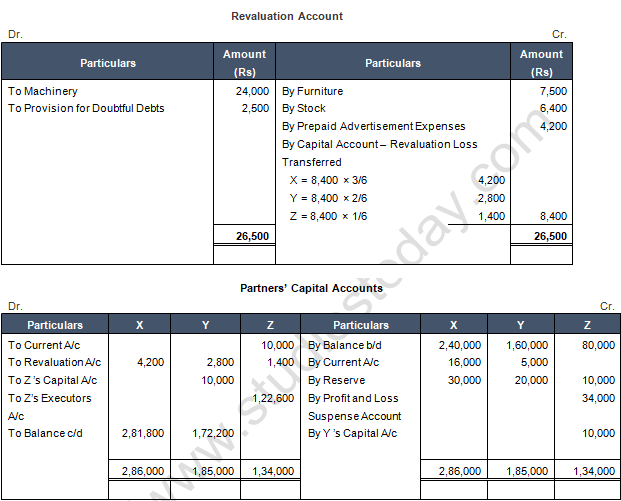Points of Knowledge:

Note1 : Calculation of New Ratio and Gaining Ratio
Old Ratio of X:Y;Z = 3:2:1
New Ratio of X:Y = 1:1
Gaining Ratio = New Ratio – Old Ratio
X’s Gain = 1/2 - 3/6 = (6-6)/12 = 0/12
Y’s Gain = 1/2 - 2/6 = (6-4)/12 = 2/12
So Gaining Ratio of X:Y = 0:2

Note 2: Goodwill of the firm = 60,000
Z will get for goodwill from X and Y = 60,000 × 1/6 = 10,000
X will transfer for goodwill to Z = 10,000 × 0/2 = 0

Note 3: Share of profit of Z = 8,16,000 × 1/6 × 3/12 = 34,000

Question 26:  X, Y and Z are partners in a firm sharing profits and losses in the ratio of 5 : 3 : 2 . Their Balance Sheet as at 31st March, 2018 was as follows: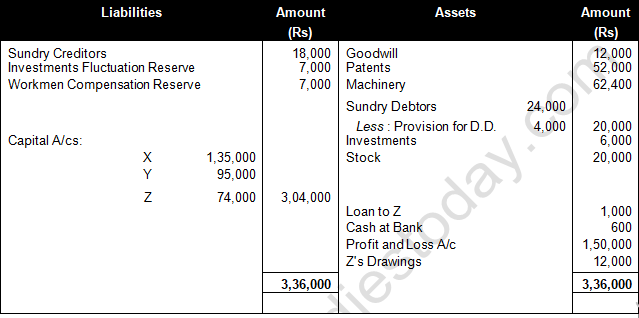Z died on 1st April, 2018, X and Y decide to share future profits and losses in ratio of 3 : 5 . It was agreed that:

(i)      Goodwill of the firm be valued 212/212 years' purchase of average of four completed years' profits which were: 2014-15——Rs 1,00,000; 2015-16——Rs 80,000; 2016-17——Rs 82,000.

(ii)     Stock undervalued by Rs 14,000 and machinery overvalued by Rs 13,600.

(iii)    All debtors are good. A debtor whose dues of Rs 400 were written off as bad debts paid 50% in full settlement.

(iv)    Out of the amount of insurance premium which was debited entirely to Profit and Loss Account, Rs 2,200 be carried forward as an unexpired insurance premium.

(v)     Rs 1,000 included in Sundry Creditors is not likely to arise.

(vi)    A claim of Rs 1,000 on account of Workmen Compensation to be provided for.

(vii)   Investment be sold for Rs 8,200 and a sum of Rs 11,200 be paid to execution of immediately. The balance to be paid in four equal half-yearly instalments together with interest @ 8% p.a. at half year rest.
Show Revaluation Account, Capital Accounts of Partners and the Balance Sheet of the new firm.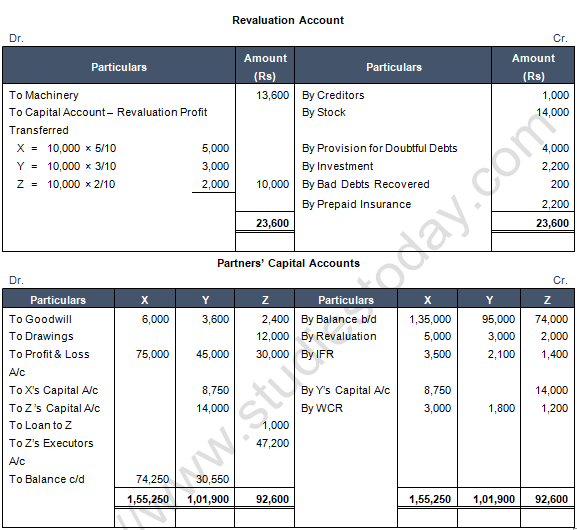Points of Knowledge :

Note 1: Calculation of New Ratio and Gaining Ratio:
Old Ratio of X:Y:Z = 5:3:2
New Ratio of X:Y = 3:5
Gaining Ratio = New Ratio – Old Ratio
X’s Gain = 3/8 - 5/10 = (30-40)/80 = - 10/80 (Sacrifice)
Y’s Gain = 5/8 - 3/10 = (50-24)/80 = 26/80

Note 2: Goodwill of the firm = Average profit × Years Purchase
= ( (1,00,000+80,000+82,000-1,50,000)/4) × 2.5 years purchase
= 28,000 × 2.5 years purchase = 70,000
Z will get for goodwill from Y = 70,000 × 2/10 = 14,000
X will get for goodwill from Y = 70,000 × 10/80 = 8,750
Y will transfer for goodwill to X and Z = 70,000 × 26/80 = 22,750
Condition for goodwill treatment: Gaining partner Y to Sacrificing partner X and Z.

Question 27:  XY and Z were partners in a firm sharing profits in the ratio of 2 : 2 : 1 . On 31st March, 2018 , their Balance Sheet was as follows :Y died on 30th June, 2018. The Partnership Deed provided for the following on the death of a partner:
(i) Goodwill of the business was to be calculated on the basis of 2 times the average profit of the past 5 years. The profits for the years ended 31st March, 2018, 31st March, 2017, 31st March, 2016, 31st March, 2015 and 31st March, 2014 were Rs 3,20,000 (Loss); Rs 1,00,000; Rs 1,60,000; Rs 2,20,000 and Rs 4,40,000 respectively.

(ii) Y's share of profit or loss from 1st April, 2018 till his death was to be calculated on the basis of the profit or loss for the year ended 31st March, 2018. You are required to calculate the following:

(a) Goodwill of the firm and Y's share of goodwill at the time of his death.

(b) Y's share in the profit or loss of the firm till the date of his death.

(c) Prepare Y's Capital Account at the time of his death to be presented to his executors.Points of Knowledge:
Note 1: Calculation of New Ratio and Gaining Ratio
Old Ratio of X : Y : Z = 2 : 2 : 1
New Ratio of X : Z = 2 : 1
Gaining Ratio = New Ratio – Old Ratio
X’s Gain = 2/3 - 2/5 = (10-6)/15 = 4/15
Z’s Gain = 1/3 - 1/5 = (5-3)/15 = 2/15
So Gaining Ratio of X:Z = 4:2 = 2:1

Note 2: Calculation of Average Profit:
Average Profit = (Five Years Total Profit)/(5 Years)
= ((-3,20,000+1,00,000+1,60,000+2,20,000+4,40,000))/(5 Years) = 6,00,000/5 = 1,20,000

Note 3: Goodwill of the firm
= Average Profit × Two Years Purchase
= 1,20,000 × 2 = 2,40,000
Y will get for goodwill from X and Z = 2,40,000 × 2/5 = 96,000
X will transfer for goodwill to Y = 96,000 × 2/3 = 64,000
Z will transfer for goodwill to Y = 96,000 × 1/3 = 32,000
Condition for goodwill treatment: Remaining Partners to Dead partner

Note 4: Calculation of Profit to be given to the dead partners Representatives:
Y’ share of loss = Last Years Loss × 3/12 Months × 2/5
= 3,20,000 × 3/12 × 2/5 = 32,000